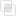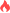Python numpy有哪些功能优于列表

Python numpy2021-09-02 09:53:142210浏览 · 0收藏 · 0评论1、与列表相比，numpy具有更自然、更方便的数学运算集成。

```a = [6, 2, 1, 4, 3]
b = [e + 1 for e in a]```

```a = np.array([6, 2, 1, 4, 3])
b = a + 1```

2、numpy也适用于每个numpy数学函数：例如，可以使用列表中每个元素的指数。

```a = [6, 2, 1, 4, 3]
b = [1, 2, 3, 2, 3]
c = [e1 + e2 for (e1, e2) in zip(a, b)]```python多行读写的操作Python修改matplotlib中的字体python如何解决黏包问题python链表的乘法问题python链表实现左移和右移Python绘图项目之海绵宝宝Python爬虫抓取名人名言网站python多线程的线程安全实现python爬虫的时候有哪些技巧可以借鉴？python如何快速建立生成器python中for语句读取生成器Python脚本如何调用外部命令python字符串转换为日期时间python计算列表项出现次数的方法js事件委托如何理解26458

js中hasOwnProperty的属性用法25676